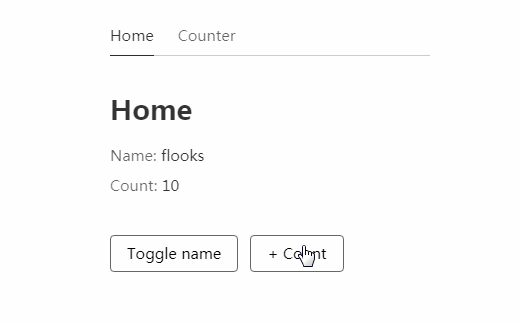/ State

# A state manager for React Hooks## flooks

A state manager for React Hooks. Maybe the simplest.

## Install

``````yarn add flooks
``````

or

``````npm install flooks
``````

## Usage

``````import { setModel, useModel } from 'flooks';

const counter = {
state: {
count: 0,
},
actions: ({ model, setState }) => ({
increment() {
const { count } = model();
setState({ count: count + 1 });
},
decrement() {
const { count } = model();
setState({ count: count - 1 });
},
async incrementAsync() {
const { increment } = model();
await new Promise((resolve) => setTimeout(resolve, 1000));
increment();
},
}),
};

setModel('counter', counter);

function Counter() {
const { count, increment, decrement, incrementAsync } = useModel('counter');
return (
<>
Count: {count}
<button onClick={increment}>+</button>
<button onClick={decrement}>-</button>
</>
);
}
``````

## API

### setModel()

``````setModel(name, model);
``````

Accepts a name string and an model object, initialize the model.

The model object needs to contain a `state` object and an `actions` function.

### useModel()

``````const { someState, someAction } = useModel(name);
``````

A React Hook. Accepts a name, returns the initialized model with all its states and actions.

### ({ model, setState }) => realActions

``````actions: ({ model, setState }) => ({
someAction() {},
});
``````

The argument of `actions` contains two functions, `model()` and `setState()`.

#### model()

``````const { someState, someAction } = model(name?);
``````

Returns the same as `useModel()`, but when get own model, `name` can be omitted.

i.e. `model()` for own model, `model('other')` for other models.

#### setState()

``````setState(payload);
``````

Used to update own model's state with the `payload` object, can't update other models'.

## FAQ

``````actions: ({ model, setState }) => ({
async someAsyncAction() {},
});
``````

When an action is async, `someAsyncAction.loading` can be use.

### Code splitting?

Supported naturally. Call `setModel()` in components, then use libraries like `loadable-components`.

### Set models together?

``````import { setModel } from 'flooks';
import a from '...';
...

const models = { a, b, c };
Object.entries(models).forEach(([name, model]) => {
setModel(name, model);
});
``````

This is not recommended. Call `setModel()` separately in components, which is more clear and flexible.

## Philosophy

1. Our philosophy is decentralization, so we recommend to bind a model and a route entry component as one module, call `setModel()` in the component to bind two.

2. No need to add a file like `store.js` or `models.js`, because no need to distribute the store from top now. Without the centralized store, just the modules consisting of components and models in the lower level.

3. A model has its own space, with `useModel()` and `model()`, all other models can be reached. Models are independent, but also connected.

4. Don't initialize a model multiple times using `setModel()`, if have a "common" model used in several places, recommend to to initialize it in an upper component, such as `App.jsx`.

5. That's all, enjoy it~

## GitHub#### React.js Examples

A nice collection of often useful examples done in React.js.

Latest
Tag